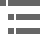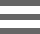## Perfect Two-Lens System CharacteristicsDuring investigations of a point source of light that does not lie in the focal plane of a lens, it is often convenient to represent a perfect lens as a system composed of two individual lens elements. This tutorial explores off-axis oblique light rays passing through such a system.

The tutorial initializes with a parallel beam of light passing through the double lens system in coincidence with the optical axis and traveling from left to right. The Tilt Angle slider can be employed to tilt the axis of the light beam through ± 25 degrees, and the Object Side Focal Length slider adjusts the focal length of the lens nearest the object between a range of 0.4 to 0.8 centimeters. The Image Side Focal Length slider can be utilized to change this value between 0.8 and 2.0 centimeters. A checkbox toggles simulations of plane and spherical wavefronts on and off, allowing the visitor to view how spherical waves are produced when a plane wavefront passes through the lens system. The blue Reset button is utilized to re-initialize the tutorial.

In the dual-lens system illustrated in the tutorial window, a spherical wavefront emanating from light source point S(1), and located at a distance δ from the optical axis of the lens, is converted by Lens(a) into a plane wave. As it exits from Lens(a), the plane wave is tilted with respect to the lens axis by an angle α. Both δ and α are related by the sine equation, with the value for f being replaced by f(a):

where f is the focal length of the perfect lens. After passing through the second lens (Lens(b)), the plane wave is converted back into a spherical wave having a center located atS(2). The result is that a perfect lens, which equals Lens(a) + Lens(b), focuses light from point S(1) onto point S(2) and also performs the reverse action by focusing light from pointS(2) onto point S(1). Focal points having such a relationship in a lens system are commonly referred to as conjugate points.

In the nomenclature of classical optics, the space between light source S(1) and the entrance surface of the first lens is referred to as the object space, while the region between the second lens exit surface and point S(2) is known as the image space. All points involved with primary or secondary light rays are termed objects (or specimens in optical microscopy), while the regions containing light rays concentrated by refraction by the lens are called images. If the light waves themselves intersect, the image is real, whereas if only the projected extensions of refracted light rays intersect, a virtual image is formed by the lens system.

If the point S(1) is expanded into a series of points spread throughout the same focal plane, then a perfect lens will focus each point in the series onto a conjugate point in the focal plane of S(2). In the case where a point set of S(1) lies in a plane perpendicular to the optical axis of the lens, then the corresponding conjugate points in set S(2) would also lie in a plane that is perpendicular to the axis. The reverse is also true: the lens will focus every point in the setS(2) onto a conjugate point on the plane or surface of point set S(1). Corresponding planes or surfaces of this type are known as conjugate planes.

### Contributing Authors

Matthew Parry-Hill and Michael W. Davidson - National High Magnetic Field Laboratory, 1800 East Paul Dirac Dr., The Florida State University, Tallahassee, Florida, 32310.

# Microscopy U - The source for microscopy education•••